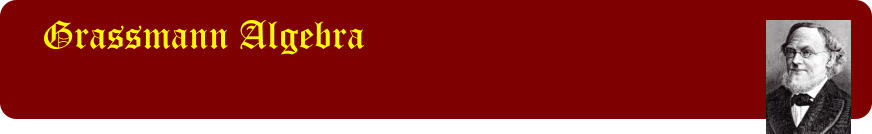Book Volume 1- Table of Contents: page 3 of 3
Understanding the scope of the printed work Volume 1 This page is an extract from “Grassmann Algebra Volume 1: Foundations - Exploring extended vector algebra with Mathematica” by John Browne. First Edition 2012.## 372

### 373

Definition of the inner product 373 Forms of the inner product 373 Definition of the interior product 374 Left and right interior products 375 Implications of the regressive product axioms 376 Orthogonality 378 Example: The interior product of a simple bivector with a vector 379

### 381

Implications of the complement axioms 381 Extended interior products 383 The precedence of the interior product 383 Converting interior products to exterior and regressive products 385 The complement form of interior products 386

### 387

The Interior Common Factor Formula 387 The Interior Common Factor Theorem 387 Examples of the Interior Common Factor Theorem 389 The computational form of the Interior Common Factor Theorem 390 Converting interior products to inner and scalar products 392

### 393

The symmetry of the inner product 393 The inner product of complements 393 The inner product as a determinant 394 Calculating inner products 394 Inner products of basis elements 396

### 396

The definition of measure 396 Unit elements 397 Calculating measures 398 The measure of vectorial elements 399 The measure of orthogonal elements 400 The measure of bound elements 401 Determining the m-vector of a bound m-vector 403 Exploring a bound measure 404

### 405

The metric tensor as a tensor of inner products 405 Induced metric tensors 406 Converting to metric elements 407 Displaying induced metric tensors as a matrix of matrices 407 Calculating induced metric tensors 408

### 409

The basic Interior Product Formula 409 Deriving Interior Product Formulae 410 Deriving Interior Product Formulae automatically 413 Exploring the computable form of the Interior Product Formula 414 The computable form of the Interior Product Formula 415 Comparing derivations of the Interior Product Formulae 416 The invariance of Interior Product Formula 417 An alternative form for the Interior Product Formula 418 Interior Product Formula B 418 The Orthogonal Decomposition Formula 420 Orthogonal Decomposition Formula B 421 Interior Product Formulae as double sums 423 Extended Interior Product Formulae 424 Complementary forms for Decomposition Formulae 427 Complementary forms for Interior Product Formulae 428 Interior Product Formulae for 1-elements 431

### 433

Introduction 433 Interior products involving the origin 434 Interior products involving points 435 Special cases 437 Formula summary for points and bound vectors 438

### 440

Defining a generalized cross product 440 Cross products involving 1-elements 440 Implications of the axioms for the cross product 441 The cross product as a universal product 443 Cross product formulae 444

### 445

The triangle components 445 The measure of the triangular components 447 Complementary forms for the triangle components 448 Expanding the components 448 Expanding the components in orthogonal factors 450 The triangle formulae for a bivector 452 The triangle formulae for a point and an m-vector 454 Special cases of the triangle formulae for a point and an m-vector 456 The orthogonal decomposition of an m-element by a 1-element 457 The measure of the triangular m-element components 458 The triangle formulae for a vector and a bound m-vector 459 Examples of the triangle formulae for a bound m-vector 461

### 462

The angle between 1-elements 462 The angle between a 1-element and a simple m-element 463 Computing angles 464 The angle between a vector and a bivector 465 The angle between bivectors in a 3-space 466 The volume of a parallelepiped 468 The angle between a point and a simple m-vector 469 The angle between simple elements 469 The angle between a bivector and an m-vector 472 Example: The angle between a bivector and a trivector 475

### 476

Introduction 476 Orthogonal decomposition 477 The decomposition formula applied 479 OrthogonalDecompose 480 Decomposition components 481 Relationships amongst the components 483 Invariance of the decomposition 486

### 487

Orthogonalizing the factors of a simple m-element 487 Orthogonalization in terms of the original factors 489 Example: Orthogonalization of a 3-element 490 The magnitude of the orthogonal factors 491 Explicit orthogonalization 492 The Zero Interior Sum Theorem 495 Composing interior sums 496

## 498

Orthogonal projection of a 1-element 498 Orthogonal projection from projective-space projection 499 Orthogonal projection of a k-element 500 Orthogonal projection onto a bivector 500 Orthogonal projection onto a trivector 502 The projection of a bound m-vector onto an m-vector 503

### 504

The shortest distance between two multiplanes 504 Decomposition of the parallel component 505 Determining the common normal 506 The closest approach of a point to a multiplane 507 The closest approach of a line to a plane 508 The common normal of two lines 508 The common normals of two parallel lines 510 The common normals of two intersecting lines 510 The common normal of two lines in three dimensions 511 The common normal of two lines from first principles 512

## 516

### 516

Biographical sources 516 A Brief Biography of Hermann Grassmann 516

### 520

Symbol types 520 Symbol forms 521 Operations 522 Special objects 523 Declarations 523 Spaces 523 Basis elements 524 Compositors 524

### 539

A note on sources to Grassmann's work 539 Bibliography 540© John Browne 2018.